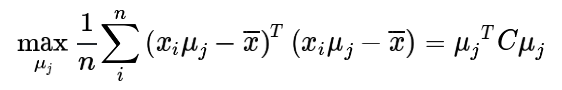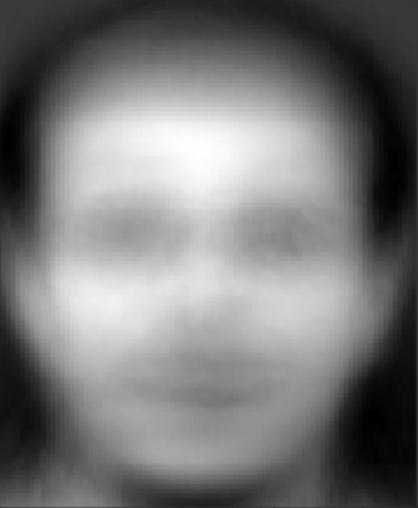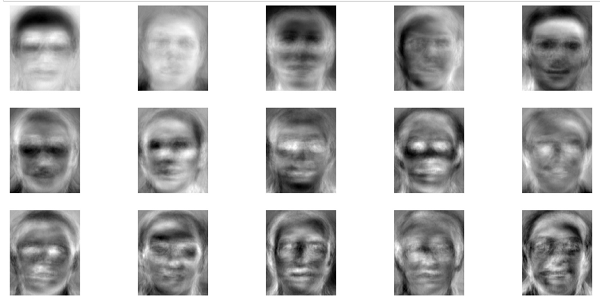# Community

Blog Events Webinars Tutorials Forum
×
Community Blog Training Facial Recognition Algorithms with Alibaba's Mars

# Training Facial Recognition Algorithms with Alibaba's Mars

This article shows you how to use Alibaba's open source Mars to implement facial recognition algorithms.

On January 16, 2019, Alibaba published the open source code of its first scientific computing engine, Mars, which is derived from MaxCompute.

Mars is a matrix-based and universally distributed computing framework. The previous articles have described what Mars is and distributed execution in Mars, as well as introduced the source code on GitHub. After reading the introduction to Mars, you may ask, "what can you do with Mars?" A complete answer to this question depends on what you want to do. Mars, as a underlying operation library, has implemented 70% of the common NumPy interfaces. This article shows how to use Mars do what you want to do.

## Singular Value Decomposition (SVD)

As a data processor, when you process massive amounts of complex data, the first thing that you may think of is the dimensional reduction. SVD is one common technique used to reduce the dimensionality. The SVD technique is provided in the `numpy.linalg` module. For example, to process 20,000 pieces of data with 100 dimensions, invoke the SVD interface:

``````In : import numpy as np

In : a = np.random.rand(20000, 100)

In : %time U, s, V = np.linalg.svd(a)
CPU times: user 4min 3s, sys: 10.2 s, total: 4min 13s
Wall time: 1min 18s``````

As we can see, even if MKL is used in NumPy, it still takes around 1 minute to run the task. As the data volume increases, the memory of a single machine no longer meets the processing requirement.

SVD is also implemented in Mars. However, SVD in Mars is faster than in NumPy, because the matrix chunk algorithm in Mars supports parallel computing:

``````In : import mars.tensor as mt

In : a = mt.random.rand(20000, 100, chunk_size=100)

In : %time U, s, V = mt.linalg.svd(a).execute()
CPU times: user 5.42 s, sys: 1.49 s, total: 6.91 s
Wall time: 1.87 s``````

The preceding result shows that Mars implements the dimensional reduction on 20,000 pieces of data within about a second, tens of times faster than NumPy to reduce the dimensionality for the same amount of data. Distributed matrix operations are of great value for performing a matrix decomposition of Taobao user data.

## Principal Component Analysis (PCA)

PCA is also an important technique for the dimensionality reduction. PCA allows data to be projected in the direction where most information is retained. We can interpret the project direction from two perspectives: maximum variance and minimum projection error. Vector and feature vector matrices in the low-dimensional representation can be used to basically reconstruct the original high-dimensional vectors. The following formula is of key importance:Xi is the data of each sample and μj is the new projection direction. Our goal is to project the data in such a way that the projection variance is maximized in order to find the main features. The matrix C in the preceding formula can be represented by a covariance matrix. Of course, this requires the centralization adjustment of input samples. Let's see how NumPy uses PCA to reduce the dimensionality of a randomly generated array:

``````import numpy as np

a = np.random.randint(0, 256, size=(10000, 100))
a_mean = a.mean(axis=1, keepdims=True)
a_new = a - a_mean
cov_a = (a_new.dot(a_new.T)) / (a.shape - 1)

# Use SVD to find the first 20 feature values in the covariance matrix
U, s, V = np.linalg.svd(cov_a)
V = V.T
vecs = V[:, :20]

# Use low-dimensional feature vectors to represent the original data
a_transformed = a.dot(vecs)``````

Because randomly generated data itself has no strong features, extract the first 20 dimensions from the data with 100 dimensions and obtain the first 99% of values of the sum according to the feature value ratio.

Now let's see how a dimensionality reduction is implemented in Mars:

``````import mars.tensor as mt

a = mt.random.randint(0, 256, size=(10000, 100))
a_mean = a.mean(axis=1, keepdims=True)
a_new = a - a_mean
cov_a = (a_new.dot(a_new.T)) / (a.shape - 1)

# Use SVD to find the first 20 feature values in the covariance matrix
U, s, V = mt.linalg.svd(cov_a)
V = V.T
vecs = V[:, :20]

# Use low-dimensional feature vectors to represent the original data
a_transformed = a.dot(vecs).execute()``````

As we can see, in addition to the "import", the only difference is that Mars also invokes "`execute`" to obtain the variables of the required data. When the "eager" mode is available in the future, even "`execute`" is no longer required. Algorithms written in NumPy can be almost seamlessly converted into multi-process and distributed programs, without having to manually write MapReduce code any more.

## Facial Recognition

After the implementation of the basic algorithms, Mars can be used in practical algorithm scenarios. The best known application of PCA is facial feature extraction and facial recognition. A single facial picture has too many dimensions, and a classifier is hard to process such a picture. The famous early face recognition algorithm, Eigenface, also adopts the PCA algorithm. This section provides a simple facial recognition program example to show how Mars implements the facial recognition algorithm.

The face databases used in this article is the ORL face database, which provides 400 gray level face pictures of 40 different persons with a resolution of 92x112 pixels. In this example, we select the first picture of each picture group as our test picture and use the other nine pictures as the training set.

First, use OpenCV for Python to read all the pictures into a large matrix (360x10304), in which each row is the gray level value of each face, and a total of 360 training samples are available. Use PCA to train data: `data_mat` is the input matrix, and `k` is the dimensions to be preserved.

``````import mars.tensor as mt
from mars.session import new_session

session = new_session()

def cov(x):
x_new = x - x.mean(axis=1, keepdims=True)
return x_new.dot(x_new.T) / (x_new.shape - 1)

def pca_compress(data_mat, k):
data_mean = mt.mean(data_mat, axis=0, keepdims=True)
data_new = data_mat - data_mean

cov_data = cov(data_new)

U, s, V = mt.linalg.svd(cov_data)
V = V.T
vecs = V[:, :k]

data_transformed = vecs.T.dot(data_new)
return session.run(data_transformed, data_mean, vecs)``````

For the purpose of predictive recognition, in addition to the transformation to low-dimensional data, it is also required to return the average values and low-dimensional vector spaces. We can obtain an average face during the intermediate process, as shown below. Average faces of people in different regions drew a lot of attention several years ago. These average faces can be obtained by using this method. Here we can only see a rough face contour because the number of dimensions and samples in this example is relatively small.In fact, the features stored in `data_transformed`, if arranged by pixel, can also form the shape of a feature face This graph contains 15 feature faces, which are enough to make a human face classifier.The `session.run` function is also used in functional PCA. This is because that the three results to be returned are not mutually independent. When using delayed execution, submitting operations three times will increase computations while submitting operations at the same time will not. We are also working on improving the immediate execution mode and pruning the related graphs.

When the training is completed, we can use lower-dimensional data for facial recognition. Transform the input pictures from the original non-training samples to the dimension representation with reduced dimensionality. We use the Euclidean distance to determine the difference from each piece of facial data in the previous training samples. The smallest distance indicates successfully recognized faces. Of course a threshold can be set, and facial recognition fails if the smallest value exceeds the threshold. The final accuracy rate for this dataset is 92.5%, meaning that a simple face recognition algorithm has been built successfully.

``````# Find the Euclidean distance
def compare(vec1, vec2):
distance = mt.dot(vec1, vec2) / (mt.linalg.norm(vec1) * mt.linalg.norm(vec2))
return distance.execute()``````

## Future Work

The preceding sections describe how to use Mars to implement facial recognition algorithms. We can see that Mars provides NumPy-like interfaces that are very friendly for algorithm developers to use. You don't have to worry when the algorithm scale exceeds the processing capacity of a single machine. In the distributed environment, Mars processes all parallel logic for you.

Of course, many aspects of Mars still needs to be improved. For example, operating on feature values and feature vectors when using PCA for a factorization of a covariance matrix. This enables a much smaller number of computations than SVD. However, currently the linear algebra module has not implemented methods to compute feature vectors. We will gradually add support for these features, including the implementation of various upper-layer algorithm interfaces in SciPy. Feel free to raise issues on GitHub and help us improve upon Mars.

Mars is a newly released open source project. We hope you can join us in continuously improving Mars.

0 0 0
Share on

# Related Products

• ##Quick Starts

Deploy custom Alibaba Cloud solutions for business-critical scenarios with Quick Start templates.

• ##ApsaraDB for HBase

ApsaraDB for HBase is a NoSQL database engine that is highly optimized and 100% compatible with the community edition of HBase.

• ##MaxCompute

Conduct large-scale data warehousing with MaxCompute

• ##Starter Package

Easy-to-use high-performance virtual machines with data transfer plan starting from \$2.50 a month